# Tableau Calculated fields

Rating: 5

6014

As the data analysis and visualization with tableau become more complex we need data that are not present in our originals data sources. Sometimes we need to visualize data from existing data sources and we need to create that field. One of the most important features of tableau is to give users the power to create additional fields from existing dimensions and measures.

Calculated fields play an important role in data visualization in tableau. These are fields that we create that don’t already exist in your data source. These calculated fields are created using formulas that are often based on other fields.  Within calculated fields we can define hard coded constants, can do simple mathematical operations, use more complex mathematical formulas, create new conditional categories and do type conversions, and much more. Calculated fields are very similar to excel formulae.

Accelerate Your career with Tableau Training and become expertise in Tableau Enroll For Free  "Tableau Training" Demo!

Once defined, Calculated Fields are available across the entire workbook as long as the worksheets are using the same data source. You can use Calculated Fields in the same way you are using the dimensions and measures from your source data.

## Tableau Calculated Field in List

### Elements of calculated fields

Calculated fields have the following elements.

• Fields: These consist of the existing fields in your data source and can include other calculated fields and sets. In formulas, fields appear in orange text.
• Functions: These are built-in operations that can be performed on data, such as COUNT, DATETIME, SUM, FIND, and DAY. Functions appear as light blue text in formulas.

• Operators: These include the standard mathematical operators like +, –, and *. Operators show up as black text in formulas.

• Parameters: These are placeholder variables that you can use in a formula so that the actual value is specified at runtime. Parameters appear as purple text in formulas.

### Creating a calculated field

Let’s get started with creating a calculated filed. We will use the superstore sample data sheets that is included with tableau. (tableau 10.3 desktop edition)

1. Connect to the sample data superstore and create a new worksheet.

2. Click the drop down to the right of Dimensions on the Data pane and select Create Calculated Field to open the calculation editor.

3. Name a new field ‘Discount Ratio’ and create the following formula:

IIF([Sales] !=0, [Discount]/[Sales],0)

IIF is used to perform logical tests and return appropriate values. Here we are calculating discount ratio on sales using existing fields sales and discount. If sales are not zero, then then the discount ratio will be discount divided by sales. The first parameter [Sales] !=0 is the test condition, the second parameter [Discount]/[Sales] is the result and third parameter is the result if the condition fails. Here it is given zero.

4. Click OK to add the new field to the Measures area in the Data pane. The new field is listed under Measures instead of Dimensions because the calculation returns a number.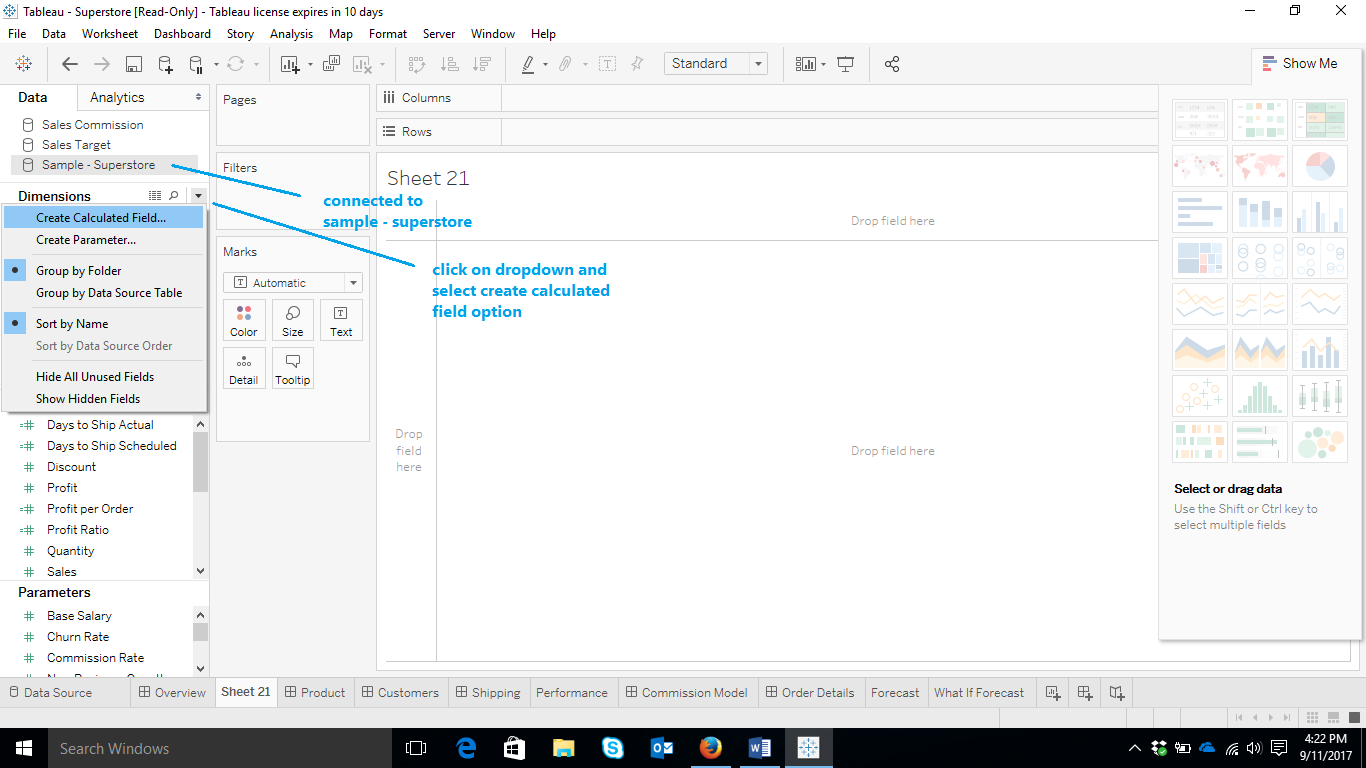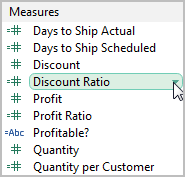5. Use the calculation in a new view.

### Edit the calculated field

1. To edit Right-click Discount Ratio in the Data pane and select Edit.

2. In the calculation editor, change the formula to compute discount ratio only for sales over \$2000:

IIF([Sales] > 2000, [Discount]/[Sales], 0)

3. Click ok to update the view automaticallyCheckout Tableau Interview Resumes

### Drag and drop calculated field

1. First create or edit a field. Open the calculation editor.

2. Drag all or part of the formula to a shelf, dropping it on an existing field, to see how it changes the view.

3. Double-click the field you just dropped on the shelf to open it as an ad-hoc calculation. Make changes to ad-hoc calculation.

4. Drag the ad-hoc calculation back to the calculation editor and drop it on the original formula in the calculation editor, thereby replacing the original formula.

The calculation editor has built-in coloring and validation to help you avoid syntax errors. As you create a formula, syntax errors are underlined with a red line. Hover over the error to see a suggestion for resolving it.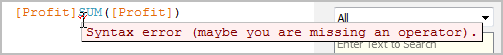Example: Calculating the average order value (AOV)

To find out average order value we have to find out the total number of orders and divide it with total sales.

The total number of orders doesn’t present in original data source. So let’s figure it out. Create a calculated field and name it ‘orders’. The formula to get the distinct numbers is COUNTD.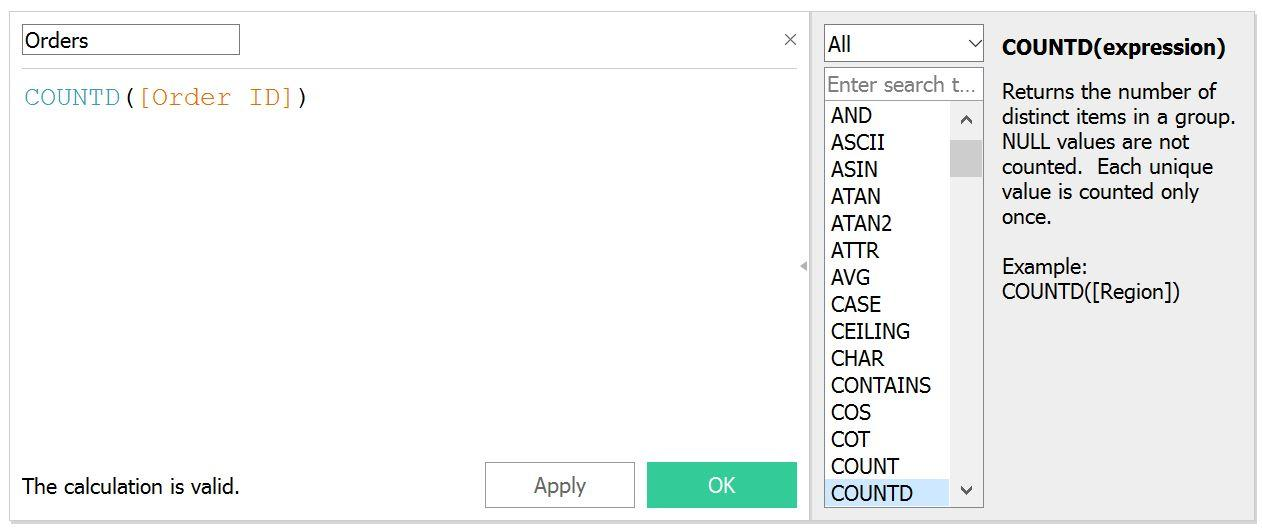Now we have to find out the AOV. We have sales and now we have the total number of order. Now create a calculated field name it AOV. AOV can be calculated by dividing sales by total order.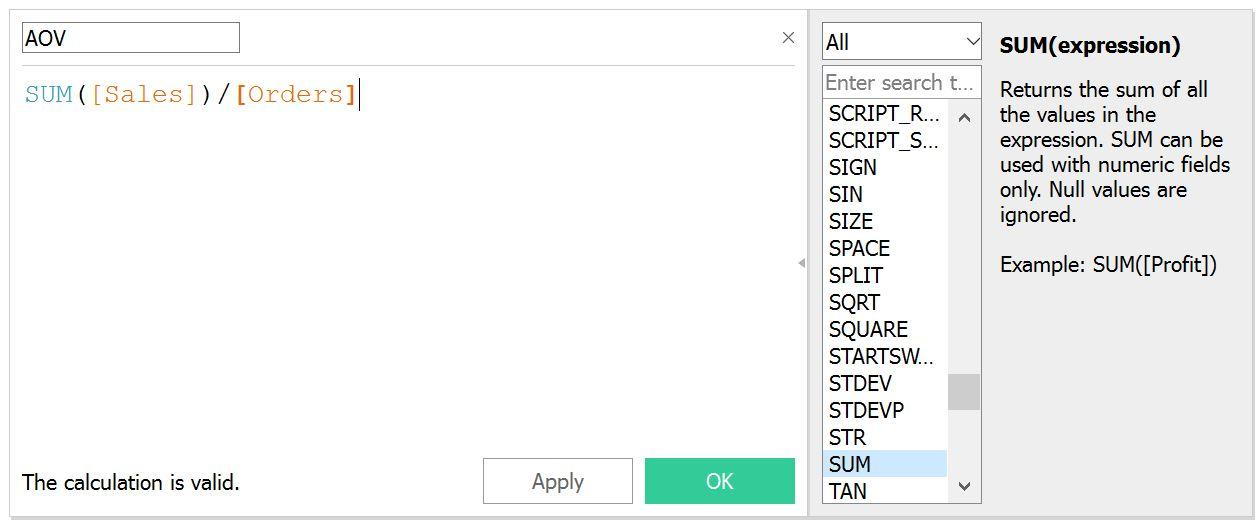Now place the measures in the worksheet and we will get the visualization.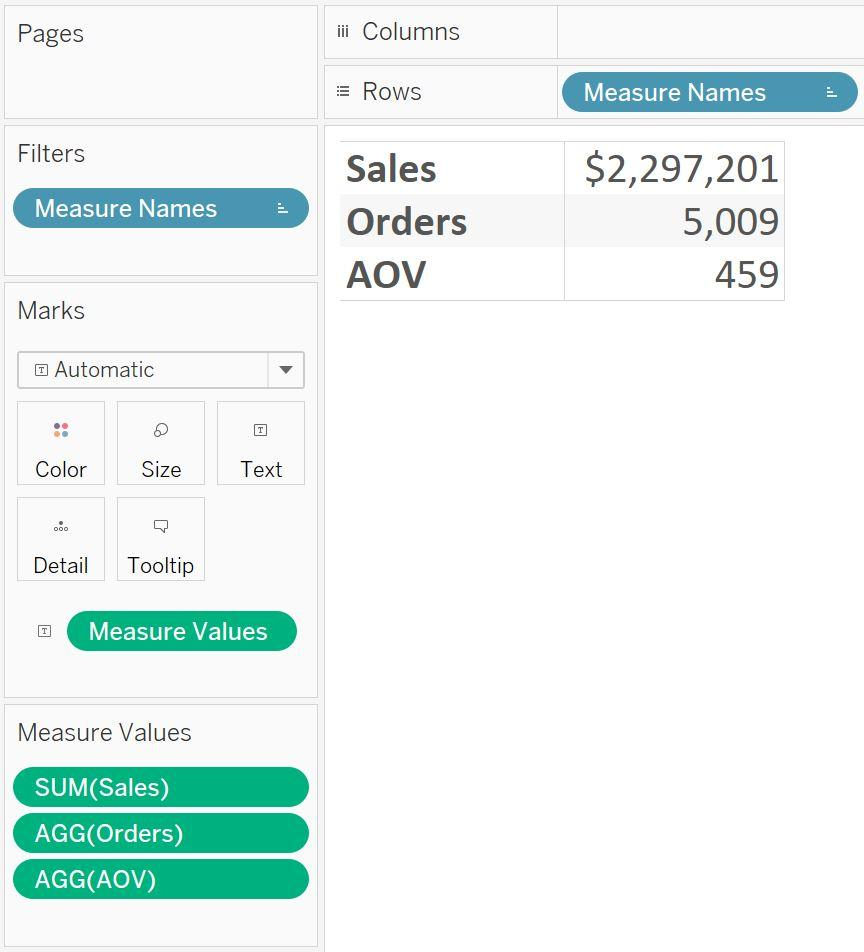The AOV returns a simple number without formatting. To change it to the currency we can click the format number option in default properties in a calculated field.

Explore Splunk Sample.

### List Of Tableau Courses:Course Schedule
NameDates
Tableau TrainingJan 31 to Feb 15
Tableau TrainingFeb 04 to Feb 19
Tableau TrainingFeb 07 to Feb 22
Tableau TrainingFeb 11 to Feb 26
Last updated: 29 January 2023